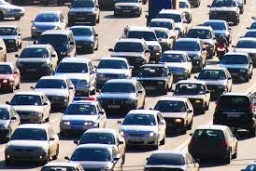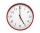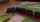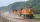# Deceleration of car

The car has a speed of 60 km/h and after 100 m journey speed of 40 km/h. What is the deceleration of a car if we assume that its movement is constantly slowed down?

a =  0.7716 m/s2

### Step-by-step explanation:Did you find an error or inaccuracy? Feel free to write us. Thank you!Tips to related online calculators
Do you have a linear equation or system of equations and looking for its solution? Or do you have a quadratic equation?
Do you want to convert length units?
Do you want to convert velocity (speed) units?
Do you want to convert time units like minutes to seconds?

## Related math problems and questions:

• Car crashOn the road, with a maximum permitted speed of 60 km/h, there was a car crash. From the length of the vehicle's braking distance, which was 40 m, the police investigated whether the driver did not exceed that speed. What is the conclusion of the police, a
• CarThe driver of a car is to get to 608 km distant city. From atlas found that 162 km will have to pass through the cities at average speed 48 km/h. Remainder of the journey pass outside the cities at average speed 116 km/h. Calculate how many hours it will
• Brakes of a carFor the brakes of a passenger car to be effective, it is prescribed that a car moving on a horizontal road at a speed of 40 km. A car must stop on the track 15.4 m. What is the deceleration of the car?
• DriverThe car driver drives at a speed of 100 km/h, saw the obstacle, and began to brake with a slowing of 5 m/s². What is the length of the pathway to stopping the car when the driver has registered the obstacle with a delay of 0.7 s?
• G forcesCalculate deceleration of car (as multiple of gravitational acceleration g = 9.81 m/s2) occurs when a vehicle in a frontal collision slows down uniformly from a speed 111 km/h to 0 km/h in 1.2 meters trajectory.
• Braking distanceThe car travels at an average speed of 12 km/h and detects an obstacle 10 m in front of it. At 1 m in front of the obstacle it already runs 2 km/h. What is the braking distance? What is the required deceleration for stop in: A) 1m B) 1s?
• The carThe car weight 1280 kg, increased its speed from 7.3 m/s to 63 km/h on a track of 37.2 m. What force did the car engine have to exert?
• BrakesThe braking efficiency of a passenger car is required to stop at 12.5 m at an initial speed of 40 km/h. What is the acceleration braking by brakes?
• Acceleration 2if a car traveling at a velocity of 80 m/s/south accelerated to a velocity of 100 m/s east in 5 seconds, what is the cars acceleration? using Pythagorean theorem
• AccelerationThe car accelerates at rate 0.5m/s2. How long travels 400 meters and what will be its speed?
• Two citiesThe car goes from city A to city B at an average speed of 70 km/h, back at an average speed of 50 km/h. If it goes to B and back at an average speed of 60 km/h, the whole ride would take 8 minutes less. What is the distance between cities A and B?
• Two carsTwo cars started against each other at the same time to journey long 346 km. The first car went 53 km/h and the second 53 km/h. What distance will be between these cars 20 minutes before meet?
• CollisionThe two bodies, whose initial distance is 240 m, move evenly against each other consistently. The first body has an initial velocity of 4 m/s and an acceleration of 3 m/s2, the second body has an initial speed of 6 m/s and an acceleration of 2 m/s2. Fin
• An accelerationThe car goes on a straight road at a speed of 72 km/h. At some point, the driver starts to brake and stops the car in 5 seconds. Find: (a) the acceleration during braking (b) the distance traveled during braking.
• Express trainInternational express train drove from Kosice to Teplice. In the first 279 km, the track was repaired, and therefore it was moving at a speed of 10km/h less than it was scheduled to drive. The rest of the 465 km trip has increased the speed by 8 km/h than
• Train speedThe train speed is decreased during 50 sec from 72 km/h to 36 km/h. Assuming that the train movement is equally slowing, find the the acceleration and the distance that it travels at.
• AbyssThe stone fell into the abyss: 2 seconds after we heard hitting bottom. How deep is the abyss (neglecting air resistance)? (gravitational acceleration g = 9.81 m/s2 and the speed of sound in air v = 343 m/s)